# An Overview of Naming Organic Molecules

$$\newcommand{\vecs}{\overset { \rightharpoonup} {\mathbf{#1}} }$$ $$\newcommand{\vecd}{\overset{-\!-\!\rightharpoonup}{\vphantom{a}\smash {#1}}}$$$$\newcommand{\id}{\mathrm{id}}$$ $$\newcommand{\Span}{\mathrm{span}}$$ $$\newcommand{\kernel}{\mathrm{null}\,}$$ $$\newcommand{\range}{\mathrm{range}\,}$$ $$\newcommand{\RealPart}{\mathrm{Re}}$$ $$\newcommand{\ImaginaryPart}{\mathrm{Im}}$$ $$\newcommand{\Argument}{\mathrm{Arg}}$$ $$\newcommand{\norm}{\| #1 \|}$$ $$\newcommand{\inner}{\langle #1, #2 \rangle}$$ $$\newcommand{\Span}{\mathrm{span}}$$ $$\newcommand{\id}{\mathrm{id}}$$ $$\newcommand{\Span}{\mathrm{span}}$$ $$\newcommand{\kernel}{\mathrm{null}\,}$$ $$\newcommand{\range}{\mathrm{range}\,}$$ $$\newcommand{\RealPart}{\mathrm{Re}}$$ $$\newcommand{\ImaginaryPart}{\mathrm{Im}}$$ $$\newcommand{\Argument}{\mathrm{Arg}}$$ $$\newcommand{\norm}{\| #1 \|}$$ $$\newcommand{\inner}{\langle #1, #2 \rangle}$$ $$\newcommand{\Span}{\mathrm{span}}$$$$\newcommand{\AA}{\unicode[.8,0]{x212B}}$$

This page explains how to write the formula for an organic compound given its name - and vice versa. It covers alkanes, cycloalkanes, alkenes, simple compounds containing halogens, alcohols, aldehydes and ketones.

## Background

There are two skills you have to develop in this area:

• You need to be able to translate the name of an organic compound into its structural formula.
• You need to be able to name a compound from its given formula.

The first of these is more important (and also easier!) than the second. In an exam, if you can't write a formula for a given compound, you aren't going to know what the examiner is talking about and could lose lots of marks. However, you might only be asked to write a name for a given formula once in a whole exam - in which case you only risk 1 mark.

So, we're going to look mainly at how you decode names and turn them into formulae. In the process you will also pick up tips about how to produce names yourself.

In the early stages of an organic chemistry course people frequently get confused and daunted by the names because they try to do too much at once. Don't try to read all these pages in one go. Just go as far as the compounds you are interested in at the moment and ignore the rest. Come back to them as they arise during the natural flow of your course.

## Cracking the code

A modern organic name is simply a code. Each part of the name gives you some useful information about the compound. For example, to understand the name 2-methylpropan-1-ol you need to take the name to pieces.

The prop in the middle tells you how many carbon atoms there are in the longest chain (in this case, 3). The an which follows the "prop" tells you that there aren't any carbon-carbon double bonds.

The other two parts of the name tell you about interesting things which are happening on the first and second carbon atom in the chain. Any name you are likely to come across can be broken up in this same way.

## Counting the carbon atoms

You will need to remember the codes for the number of carbon atoms in a chain up to 6 carbons. There is no easy way around this - you have got to learn them. If you don't do this properly, you won't be able to name anything!

code no of carbons
meth 1
eth 2
prop 3
but 4
pent 5
hex 6

## Types of carbon-carbon bonds

Whether or not the compound contains a carbon-carbon double bond is shown by the two letters immediately after the code for the chain length.

code means
an only carbon-carbon single bonds
en contains a carbon-carbon double bond

For example, butane means four carbons in a chain with no double bond.

Propene means three carbons in a chain with a double bond between two of the carbons.

### Alkyl groups

Compounds like methane, CH4, and ethane, CH3CH3, are members of a family of compounds called alkanes. If you remove a hydrogen atom from one of these you get an alkyl group. For example:

• A methyl group is CH3.
• An ethyl group is CH3CH2.

These groups must, of course, always be attached to something else.

## The alkanes

##### Example $$\PageIndex{1}$$: 2-methylpentane

Write the structural formula for 2-methylpentane.

###### Solution

Start decoding the name from the bit that counts the number of carbon atoms in the longest chain - pent counts 5 carbons.

Are there any carbon-carbon double bonds? No - an tells you there aren't any.

Now draw this carbon skeleton:Put a methyl group on the number 2 carbon atom:Does it matter which end you start counting from? No - if you counted from the other end, you would draw the next structure. That's exactly the same as the first one, except that it has been flipped over.Finally, all you have to do is to put in the correct number of hydrogen atoms on each carbon so that each carbon is forming four bonds.If you had to name this yourself:

• Count the longest chain of carbons that you can find. Don't assume that you have necessarily drawn that chain horizontally. 5 carbons means pent.
• Are there any carbon-carbon double bonds? No - therefore pentane.
• There's a methyl group on the number 2 carbon - therefore 2-methylpentane. Why the number 2 as opposed to the number 4 carbon? In other words, why do we choose to number from this particular end? The convention is that you number from the end which produces the lowest numbers in the name - hence 2- rather than 4-.
##### Example $$\PageIndex{2}$$: 2,3-dimethylbutane

Write the structural formula for 2,3-dimethylbutane.

###### Solution

Start with the carbon backbone. There are 4 carbons in the longest chain (but) with no carbon-carbon double bonds (an).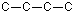This time there are two methyl groups (di) on the number 2 and number 3 carbon atoms.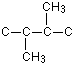Completing the formula by filling in the hydrogen atoms gives: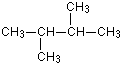##### Example $$\PageIndex{3}$$: 2,2-dimethylbutane

Write the structural formula for 2,2-dimethylbutane.

###### Solution

This is exactly like the last example, except that both methyl groups are on the same carbon atom. Notice that the name shows this by using 2,2- as well as di. The structure is worked out as before: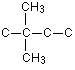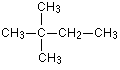##### Example $$\PageIndex{4}$$: 3-ethyl-2-methylhexane

Write the structural formula for 3-ethyl-2-methylhexane.

###### Solution

hexan shows a 6 carbon chain with no carbon-carbon double bonds.This time there are two different alkyl groups attached - an ethyl group on the number 3 carbon atom and a methyl group on number 2.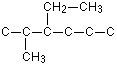Filling in the hydrogen atoms gives:If you had to name this yourself:

How do you know what order to write the different alkyl groups at the beginning of the name? The convention is that you write them in alphabetical order - hence ethyl comes before methyl which in turn comes before propyl.

## The cycloalkanes

In a cycloalkane the carbon atoms are joined up in a ring - hence cyclo.

##### Example $$\PageIndex{5}$$

Write the structural formula for cyclohexane.

###### Solution

hexan shows 6 carbons with no carbon-carbon double bonds. cyclo shows that they are in a ring. Drawing the ring and putting in the correct number of hydrogens to satisfy the bonding requirements of the carbons gives: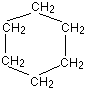## The alkenes

##### Example $$\PageIndex{6}$$: propene

Write the structural formula for propene.

###### Solution

prop counts 3 carbon atoms in the longest chain. en tells you that there is a carbon-carbon double bond. That means that the carbon skeleton looks like this:Putting in the hydrogens gives you:##### Example $$\PageIndex{7}$$: but-1-ene

Write the structural formula for but-1-ene.

###### Solution

"but" counts 4 carbon atoms in the longest chain and en tells you that there is a carbon-carbon double bond. The number in the name tells you where the double bond starts.

No number was necessary in the propene example above because the double bond has to start on one of the end carbon atoms. In the case of butene, though, the double bond could either be at the end of the chain or in the middle - and so the name has to code for the its position.

The carbon skeleton is:And the full structure is:Incidentally, you might equally well have decided that the right-hand carbon was the number 1 carbon, and drawn the structure as:##### Example $$\PageIndex{8}$$

Write the structural formula for 3-methylhex-2-ene.

###### Solution

The longest chain has got 6 carbon atoms (hex) with a double bond starting on the second one (-2-en).

But this time there is a methyl group attached to the chain on the number 3 carbon atom, giving you the underlying structure: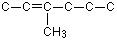Adding the hydrogens gives the final structure: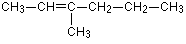Be very careful to count the bonds around each carbon atom when you put the hydrogens in. It would be very easy this time to make the mistake of writing an H after the third carbon - but that would give that carbon a total of 5 bonds.

## Compounds containing halogens

##### Example $$\PageIndex{1}$$: 1,1,1-trichloroethane

Write the structural formula for 1,1,1-trichloroethane.

###### Solution

This is a two carbon chain (eth) with no double bonds (an). There are three chlorine atoms all on the first carbon atom.##### Example $$\PageIndex{1}$$: 2-bromo-2-methylpropane

Write the structural formula for 2-bromo-2-methylpropane.

###### Solution

First sort out the carbon skeleton. It's a three carbon chain with no double bonds and a methyl group on the second carbon atom.Draw the bromine atom which is also on the second carbon.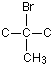And finally put the hydrogen atoms in.If you had to name this yourself:

Notice that the whole of the hydrocarbon part of the name is written together - as methylpropane - before you start adding anything else on to the name.

##### Example $$\PageIndex{1}$$: 1-iodo-3-methylpent-2-ene

Write the structural formula for 1-iodo-3-methylpent-2-ene.

###### Solution

This time the longest chain has 5 carbons (pent), but has a double bond starting on the number 2 carbon. There is also a methyl group on the number 3 carbon.Now draw the iodine on the number 1 carbon.Giving a final structure: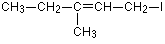## Alcohols

All alcohols contain an -OH group. This is shown in a name by the ending ol.

##### Example $$\PageIndex{1}$$: methanol

Write the structural formula for methanol.

###### Solution

This is a one carbon chain with no carbon-carbon double bond (obviously!). The ol ending shows it's an alcohol and so contains an -OH group.##### Example $$\PageIndex{1}$$: 2-methylpropan-1-ol

###### Solution

Write the structural formula for 2-methylpropan-1-ol.

The carbon skeleton is a 3 carbon chain with no carbon-carbon double bonds, but a methyl group on the number 2 carbon.The -OH group is attached to the number 1 carbon.The structure is therefore:##### Example $$\PageIndex{1}$$: ethane-1,2-diol

Write the structural formula for ethane-1,2-diol.

###### Solution

This is a two carbon chain with no double bond. The diol shows 2 -OH groups, one on each carbon atom.## Aldehydes

All aldehydes contain the group: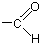If you are going to write this in a condensed form, you write it as -CHO - never as -COH, because that looks like an alcohol. The names of aldehydes end in al.

Example: propanal

Write the structural formula for propanal.

This is a 3 carbon chain with no carbon-carbon double bonds. The al ending shows the presence of the -CHO group. The carbon in that group counts as one of the chain.Example: 2-methylpentanal

Write the structural formula for 2-methylpentanal.

This time there are 5 carbons in the longest chain, including the one in the -CHO group. There aren't any carbon-carbon double bonds. A methyl group is attached to the number 2 carbon. Notice that in aldehydes, the carbon in the -CHO group is always counted as the number 1 carbon.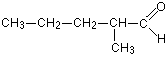## Ketones

Ketones contain a carbon-oxygen double bond just like aldehydes, but this time it's in the middle of a carbon chain. There isn't a hydrogen atom attached to the group as there is in aldehydes. Ketones are shown by the ending one.

Example: propanone
Write the structural formula for propanone.

This is a 3 carbon chain with no carbon-carbon double bond. The carbon-oxygen double bond has to be in the middle of the chain and so must be on the number 2 carbon.Ketones are often written in this way to emphasize the carbon-oxygen double bond.

Example: pentan-3-one

Write the structural formula for pentan-3-one.

This time the position of the carbon-oxygen double bond has to be stated because there is more than one possibility. It's on the third carbon of a 5 carbon chain with no carbon-carbon double bonds. If it was on the second carbon, it would be pentan-2-one.This could equally well be written: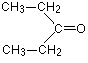This page titled An Overview of Naming Organic Molecules is shared under a CC BY-NC 4.0 license and was authored, remixed, and/or curated by Jim Clark.